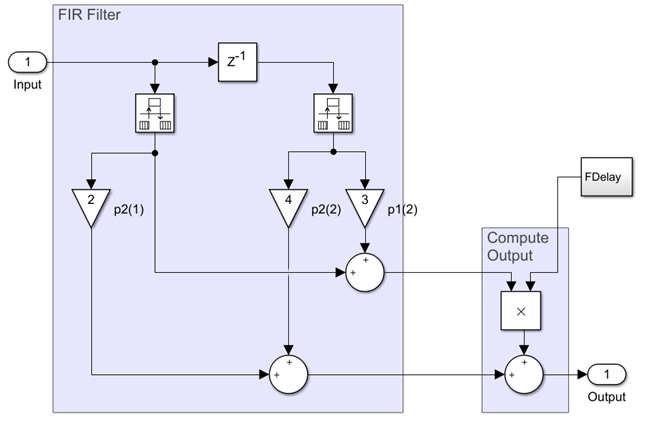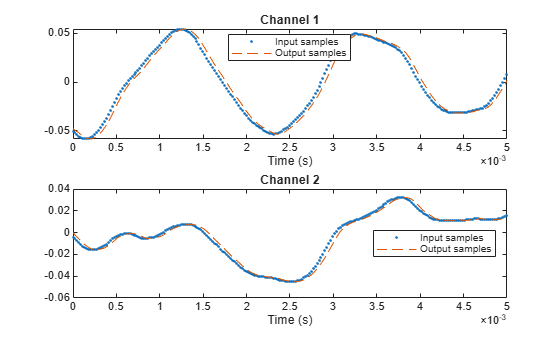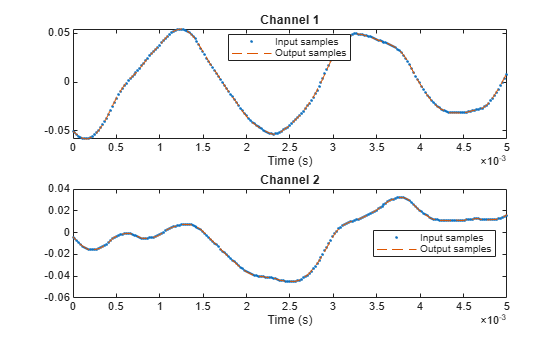# dsp.FarrowRateConverter

Polynomial sample rate converter with arbitrary conversion factor

## Description

The `dsp.FarrowRateConverter` System object™ implements a polynomial-fit sample rate conversion filter using a Farrow structure. You can use this object to convert the sample rate of a signal up or down by an arbitrary factor. This object supports fixed-point operations.

To convert the sample rate of a signal:

1. Create the `dsp.FarrowRateConverter` object and set its properties.

2. Call the object with arguments, as if it were a function.

## Creation

### Syntax

``frc = dsp.FarrowRateConverter``
``frc = dsp.FarrowRateConverter(Name,Value)``
``frc = dsp.FarrowRateConverter(fsIn,fsOut,tol,np)``

### Description

````frc = dsp.FarrowRateConverter` creates a polynomial filter-based sample rate converter System object, `frc`. For each channel of an input signal, `frc` converts the input sample rate to the output sample rate.```

example

````frc = dsp.FarrowRateConverter(Name,Value)` sets properties using one or more name-value pairs. Enclose each property name in single quotes. Example: ```frc = dsp.FarrowRateConverter('Specification','Coefficients','Coefficients',[1 2; 3 4])``` returns a filter that converts from 44.1 kHz to 48 kHz using custom coefficients that implement a 2nd-order polynomial filter.```
````frc = dsp.FarrowRateConverter(fsIn,fsOut,tol,np)` returns a sample rate converter System object, `frc`, with InputSampleRate property set to `fsIn`, OutputSampleRate property set to `fsOut`, OutputRateTolerance property set to `tol`, and PolynomialOrder property set to `np`.```

## Properties

expand all

Unless otherwise indicated, properties are nontunable, which means you cannot change their values after calling the object. Objects lock when you call them, and the `release` function unlocks them.

If a property is tunable, you can change its value at any time.

Filter Properties

Sample rate of the input signal, specified as a positive scalar in Hz. The input sample rate must be greater than the bandwidth of interest.

Data Types: `single` | `double` | `int8` | `int16` | `int32` | `int64` | `uint8` | `uint16` | `uint32` | `uint64`

Sample rate of the output signal, specified as a positive scalar in Hz. The output sample rate can represent an upsample or downsample of the input signal.

Data Types: `single` | `double` | `int8` | `int16` | `int32` | `int64` | `uint8` | `uint16` | `uint32` | `uint64`

Maximum tolerance for the output sample rate, specified as a positive scalar from 0 through 0.5, inclusive.

The actual output sample rate varies but is within the specified range. For example, if `OutputRateTolerance` is specified as `0.01`, then the actual output sample rate is in the range `OutputSampleRate` ± 1%. This flexibility often enables a simpler filter design.

Data Types: `single` | `double` | `int8` | `int16` | `int32` | `int64` | `uint8` | `uint16` | `uint32` | `uint64`

Method for specifying filter coefficients for the interpolator filter, specified as one of the following:

• `'Polynomial order'` — Use the `PolynomialOrder` property to specify the order of the Lagrange-interpolation-filter polynomial. The object calculates coefficients that meet the rate and tolerance properties.

• `'Coefficients'` — Use the `Coefficients` property to specify the polynomial coefficients directly.

Order of the Lagrange-interpolation-filter polynomial, specified as a positive integer less than or equal to 4. The object calculates coefficients that meet the rate and tolerance properties.

#### Dependencies

This property applies only when you set `Specification` to `'Polynomial order'`.

Data Types: `single` | `double` | `int8` | `int16` | `int32` | `int64` | `uint8` | `uint16` | `uint32` | `uint64`

Filter polynomial coefficients, specified as a real-valued M-by-M matrix, where M is the polynomial order.

The diagram shows the signal flow graph for a `dsp.FarrowRateConverter` object with coefficients set to ```[1 2; 3 4]```.Each branch of the FIR filter corresponds to a row of the coefficient matrix.

#### Dependencies

This property applies only when you set `Specification` to `'Coefficients'`.

Data Types: `single` | `double` | `int8` | `int16` | `int32` | `int64` | `uint8` | `uint16` | `uint32` | `uint64`

Fixed-Point Properties

Rounding method for fixed-point operations, specified as a character vector. For more information on the rounding methods see Rounding Modes.

Overflow action for fixed-point operations, specified as either `'Wrap'` or `'Saturate'`. For more details on the overflow actions, see Overflow Handling.

Data type of the filter coefficients, specified as a signed `numerictype` (Fixed-Point Designer) object. The default data type is a signed, 16-bit `numerictype` object. You must specify a `numerictype` object without specific binary-point scaling. To maximize precision, the object determines the fraction length of this data type based on the coefficient values.

Data type of the fractional delay, specified as an unsigned `numerictype` object. The default data type is an unsigned, 8-bit `numerictype` object. You must specify a `numerictype` object without specific binary-point scaling. To maximize precision, the object determines the fraction length of this data type based on the fractional delay values.

Data type of the multiplicand, specified as a signed `numerictype` object. The default data type is a signed 16-bit `numerictype` object with 13-bit fraction length. You must specify a `numerictype` object that has a specific binary point scaling.

Data type of the output, specified as one of the following:

• `'Same word length as input'` — Output word length and fraction length are the same as the input.

• `'Same as accumulator'` — Output word length and fraction length are the same as the accumulator.

• `numerictype` object — Signed fixed-point output data type. If you do not specify a fraction length, the object computes the fraction length based on the input range. The object preserves the dynamic range of the input.

## Usage

### Syntax

``y = frc(x)``

### Description

example

````y = frc(x)` resamples input `x` to create output `y` according to the rate conversion defined by `frc`.```

### Input Arguments

expand all

Input signal, specified as a vector or matrix. The row length of `x` must be a multiple of the overall decimation factor. Each column of `x` is treated as a separate channel.

### Output Arguments

expand all

Resampled signal, returned as a vector or matrix.

## Object Functions

To use an object function, specify the System object as the first input argument. For example, to release system resources of a System object named `obj`, use this syntax:

`release(obj)`

expand all

 `getPolynomialCoefficients` Get polynomial coefficients of farrow rate conversion filter `getActualOutputRate` Get actual output rate `getRateChangeFactors` Get overall interpolation and decimation factors `generatehdl` Generate HDL code for quantized DSP filter (requires Filter Design HDL Coder)
 `freqz` Frequency response of discrete-time filter System object `fvtool` Visualize frequency response of DSP filters `info` Information about filter System object `cost` Estimate cost of implementing filter System object
 `step` Run System object algorithm `release` Release resources and allow changes to System object property values and input characteristics `reset` Reset internal states of System object

## Examples

collapse all

Note: If you are using R2016a or an earlier release, replace each call to the object with the equivalent `step `syntax. For example, `obj(x) `becomes `step(obj,x)`.

Note: The `dsp.AudioFileWriter` System object™ is not supported in MATLAB Online.

Create a `dsp.FarrowRateConverter` System object™ to convert an audio signal from 44.1 kHz to 96 kHz. Set the polynomial order for the filter.

```fs1 = 44.1e3; fs2 = 96e3; LagrangeOrder = 2; % 1 = linear interpolation frc = dsp.FarrowRateConverter('InputSampleRate',fs1,... 'OutputSampleRate',fs2,... 'PolynomialOrder',LagrangeOrder); ar = dsp.AudioFileReader('guitar10min.ogg','SamplesPerFrame',14700); aw = dsp.AudioFileWriter('guitar10min_96kHz.wav','SampleRate',fs2);```

Check the resulting interpolation and decimation factors.

`[interp,decim] = getRateChangeFactors(frc)`
```interp = 320 ```
```decim = 147 ```

Display the polynomial that the object uses to fit the input samples.

`coeffs = getPolynomialCoefficients(frc)`
```coeffs = 3×3 0.5000 -0.5000 0 -1.0000 0 1.0000 0.5000 0.5000 0 ```

Convert 100 frames of the audio signal. Write the result to a file.

```for n = 1:1:100 x = ar(); y = frc(x); aw(y); end```

Release the `AudioFileWriter` System object™ to complete creation of the output file.

```release(aw) release(ar)```

Plot the input and output signals of the 100th frame of data. Delay the input to compensate for the latency of the filter.

```t1 = 0:1/fs1:1/30-1/fs1; t2 = 0:1/fs2:1/30-1/fs2; delay = ceil((LagrangeOrder+1)/2)/fs1; el1 = 1:length(t1)-delay; el2 = 1:length(t2); el2(1:delay) = []; figure subplot(2,1,1) plot(t1(1:length(el1)),x(el1,1)) hold on plot(t1(1:length(el1)),x(el1,2)) xlabel('Time (s)') title('Input Channels') subplot(2,1,2) plot(t2(1:length(el2)),y(el2,1)) hold on plot(t2(1:length(el2)),y(el2,2)) xlabel('Time (s)') title('Output Channels')```Zoom in to see the difference in sample rates.

```figure subplot(2,1,1) plot(t1(1:length(el1)),x(el1,1),'o-') hold on plot(t2(1:length(el2)),y(el2,1),'d--') xlim([0.0104 0.0107]) title('First Channel') xlabel('Time (s)') legend('Input','Output') subplot(2,1,2) plot(t1(1:length(el1)),x(el1,2),'o-') hold on plot(t2(1:length(el2)),y(el2,2),'d--') xlim([0.0104 0.0107]) xlabel('Time (s)') title('Second Channel') legend('Input','Output')```Create a `dsp.FarrowRateConverter` System object™ with 0% tolerance. The output rate is equal to the `OutputSampleRate property`. The input size must be a multiple of the decimation factor, `M`. In this case M is 320.

```frc = dsp.FarrowRateConverter('InputSampleRate',96e3,'OutputSampleRate',44.1e3); FsOut = getActualOutputRate(frc) ```
```FsOut = 44100 ```
`[L,M] = getRateChangeFactors(frc) `
```L = 147 ```
```M = 320 ```

Allow a 1% tolerance on the output rate and observe the difference in decimation factor.

```frc.OutputRateTolerance = 0.01; FsOut2 = getActualOutputRate(frc) ```
```FsOut2 = 4.4308e+04 ```
`[L2,M2] = getRateChangeFactors(frc) `
```L2 = 6 ```
```M2 = 13 ```

The decimation factor is now only 13. The lower the decimation factor, the more flexibility in input size. The output rate is within the range `OutputSampleRate` $±$ 1%.

Create a `dsp.FarrowRateConverter` System object™ with default properties. Compute and display the frequency response.

```frc = dsp.FarrowRateConverter; [h,f] = freqz(frc); plot(f,20*log10(abs(h))) ylabel('Filter Response') xlabel('Frequency (rad/s)')```Create a dsp.FarrowRateConverter System object™ with default values. Determine its computational cost: the number of coefficients, number of states, multiplications per input sample, and additions per input sample.

```frc = dsp.FarrowRateConverter; cst = cost(frc)```
```cst = struct with fields: NumCoefficients: 16 NumStates: 3 MultiplicationsPerInputSample: 14.1497 AdditionsPerInputSample: 11.9728 ```

Repeat the computation, allowing for a 10% tolerance in the output sample rate.

```frc.OutputRateTolerance = 0.1; ctl = cost(frc)```
```ctl = struct with fields: NumCoefficients: 16 NumStates: 3 MultiplicationsPerInputSample: 13 AdditionsPerInputSample: 11 ```

## Algorithms

Farrow filters implement piecewise polynomial interpolation using Horner’s rule to compute samples from the polynomial. The polynomial coefficients used to fit the input samples correspond to the Lagrange interpolation coefficients.

Once a polynomial is fitted to the input data, the value of the polynomial can be calculated at any point. Therefore, a polynomial filter enables interpolation at arbitrary locations between input samples.

You can use a polynomial of any order to fit to the existing samples. However, since large-order polynomials frequently oscillate, polynomials of order 1, 2, 3, or 4 are used in practice.

The block computes interpolated values at the desired locations by varying only the fractional delay, µ. This value is the interval between the previous input sample and the current output sample. All filter coefficients remain constant.

• The input samples are filtered using M + 1 FIR filters, where M is the polynomial order.

• The outputs of these filters are multiplied by the fractional delay, µ.

• The output is the sum of the multiplication results.

 Hentschel, T., and G. Fettweis. "Continuous-Time Digital Filters for Sample-Rate Conversion in Reconfigurable Radio Terminals." Frequenz. Vol. 55, Number 5-6, 2001, pp. 185–188.

Watch now